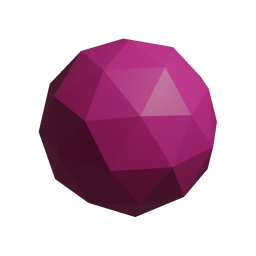## Matrices

← Back to list of classes

## Description

Summary

Classes and functions related to matrices are defined here

## Members

• ### DotProduct (AngouriMath. Entity. Matrix, AngouriMath. Entity. Matrix)

Method

Summary

Returns the dot product of two Matrixs that are matrices.

Parameter "A"

First matrix (its width is the result's width)

Parameter "B"

Second matrix (its height is the result's height)

Returns

A two-dimensional Matrix (matrix) as a result of symbolic multiplication

• ### Interval (AngouriMath. Entity, AngouriMath. Entity)

Summary

Creates a closed interval (segment)

• ### Interval (AngouriMath. Entity, System. Boolean, AngouriMath. Entity, System. Boolean)

Summary

Creates an interval with custom endings

• ### Matrix (AngouriMath. Entity[0:, 0:])

Summary

Creates an instance of Matrix that is a matrix.

Parameter "values"

A two-dimensional array of values

Returns

A two-dimensional Matrix which is a matrix

• ### Matrix (System. Int32, System. Int32, AngouriMath. Entity[])

Summary

Creates an instance of Matrix that is a matrix.
Usage example:
var t = MathS.Matrix(5, 3,
10, 11, 12,
20, 21, 22,
30, 31, 32,
40, 41, 42,
50, 51, 52);


creates 5×3 matrix with the appropriate elements

Parameter "rows"

Number of rows (first axis)

Parameter "columns"

Number of columns (second axis)

Parameter "values"

Array of values of the matrix so that its length is equal to
the product of rows and columns

Returns

A two-dimensional Matrix which is a matrix

• ### MatrixMultiplication (AngouriMath. Entity. Matrix, AngouriMath. Entity. Matrix)

Method

Summary

Returns the dot product of two Matrixs that are matrices.

Parameter "A"

First matrix (its width is the result's width)

Parameter "B"

Second matrix (its height is the result's height)

Returns

A two-dimensional Matrix (matrix) as a result of symbolic multiplication

• ### PointwiseMultiplication (AngouriMath. Entity. Matrix, AngouriMath. Entity. Matrix)

Method

Summary

Performs a pointwise multiplication operation,
or throws exception if shapes mismatch

• ### ScalarProduct (AngouriMath. Entity. Matrix, AngouriMath. Entity. Matrix)

Method

Summary

Returns the scalar product of two Matrixs that are vectors with the same length.

Parameter "a"

First vector (order does not matter)

Parameter "b"

Second vector

Returns

The resulting scalar which is an Entity and not a Matrix

• ### Vector (AngouriMath. Entity[])

Method

Summary

Creates an instance of Matrix that is a vector.

Parameter "values"

The cells of the Matrix

Returns

A one-dimensional Matrix which is a vector

2019-2021 Angouri · Project's repo · Site's repo · Octicons